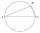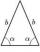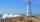Regular 5-gon

Calculate area of the regular pentagon with side 7 cm.

Result

S =  84.3 cm2

Solution:Leave us a comment of example and its solution (i.e. if it is still somewhat unclear...):Be the first to comment!Next similar examples:

1. PentagonCalculate the area of regular pentagon, which diagonal is u=17.
2. Hexagon ACalculate area of regular hexagon inscribed in circle with radius r=9 cm.
3. ISO trapeziumCalculate area of isosceles trapezoid with base 95 long, leg 27 long and with the angle between the base and leg 70 degrees.
4. SAS triangleThe triangle has two sides long 7 and 19 and included angle 36°. Calculate area of this triangle.
5. TriangleCalculate the area of right triangle ΔABC, if one leg is long 14 and its opposite angle is 59°.
6. TriangleCalculate the area of ​​the triangle ABC if b = c = 17 cm, R = 19 cm (R is the circumradius).
7. Height 2Calculate the height of the equilateral triangle with side 38.
8. Right triangleCalculate the length of the remaining two sides and the angles in the rectangular triangle ABC if a = 10 cm, angle alpha = 18°40'.
9. SinesIn ▵ ABC, if sin(α)=0.5 and sin(β)=0.6 calculate sin(γ)
10. Triangle TBCTBC is isosceles triangle with base TB with base angle 63° and legs length |TC| = |BC| = 25. How long is the base TB?
11. How farFrom the top of a lighthouse 145 ft above sea level, the angle of depression of a boat 29°. How far is the boat from the lighthouse?
12. BuildingThe building I focused at an angle 30°. When I moved 5 m building I focused at an angle 45°. What is the height of the building?
13. SteepleSteeple seen from the road at an angle of 75°. When we zoom out to 25 meters, it is seen at an angle of 20°. What is high?
14. High wallI have a wall 2m high. I need a 15 degree angle (upward) to second wall 4 meters away. How high must the second wall?
15. Center traverseIt is true that the middle traverse bisects the triangle?
16. Theorem proveWe want to prove the sentence: If the natural number n is divisible by six, then n is divisible by three. From what assumption we started?
17. TrigonometryIs true equality? ?